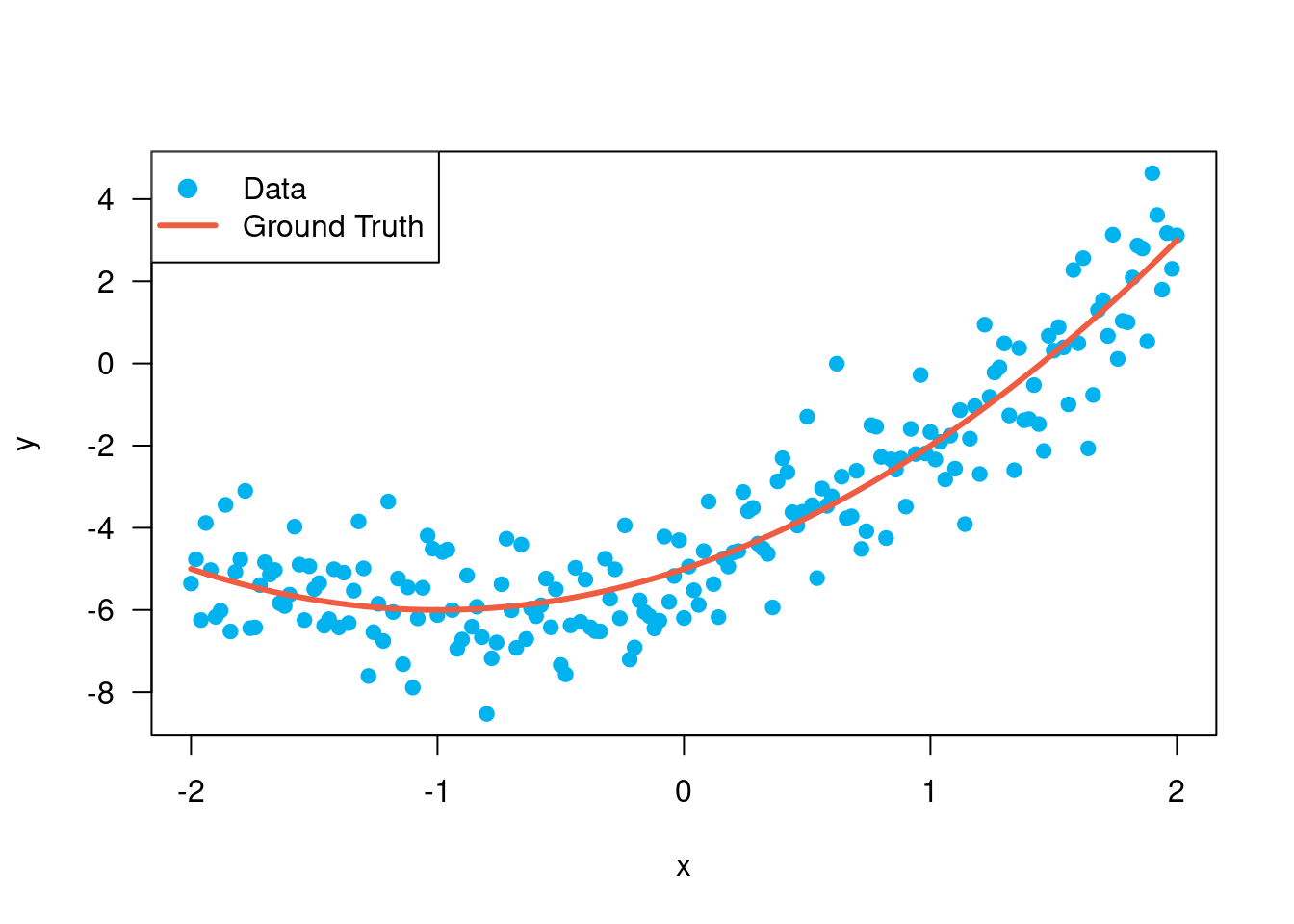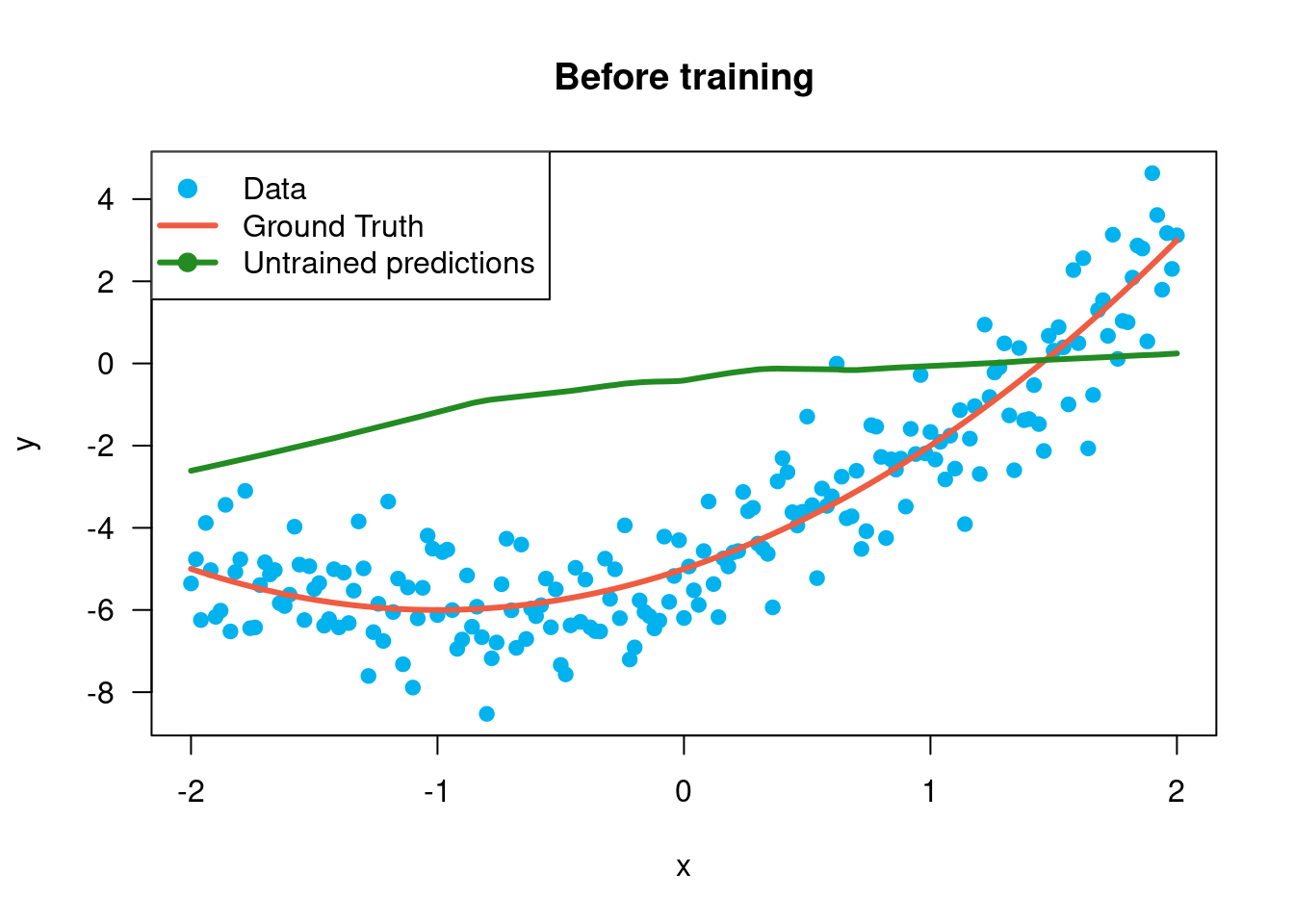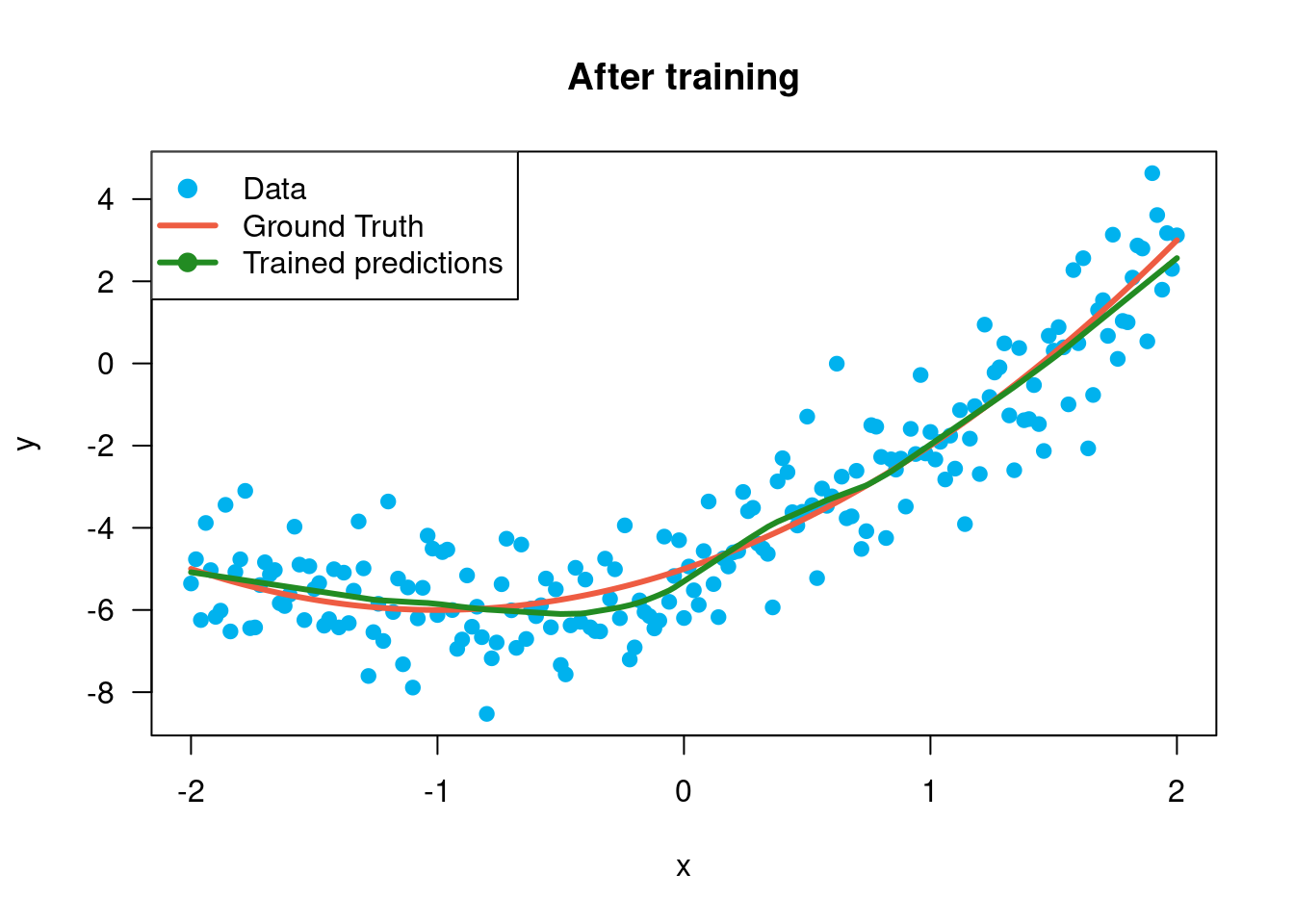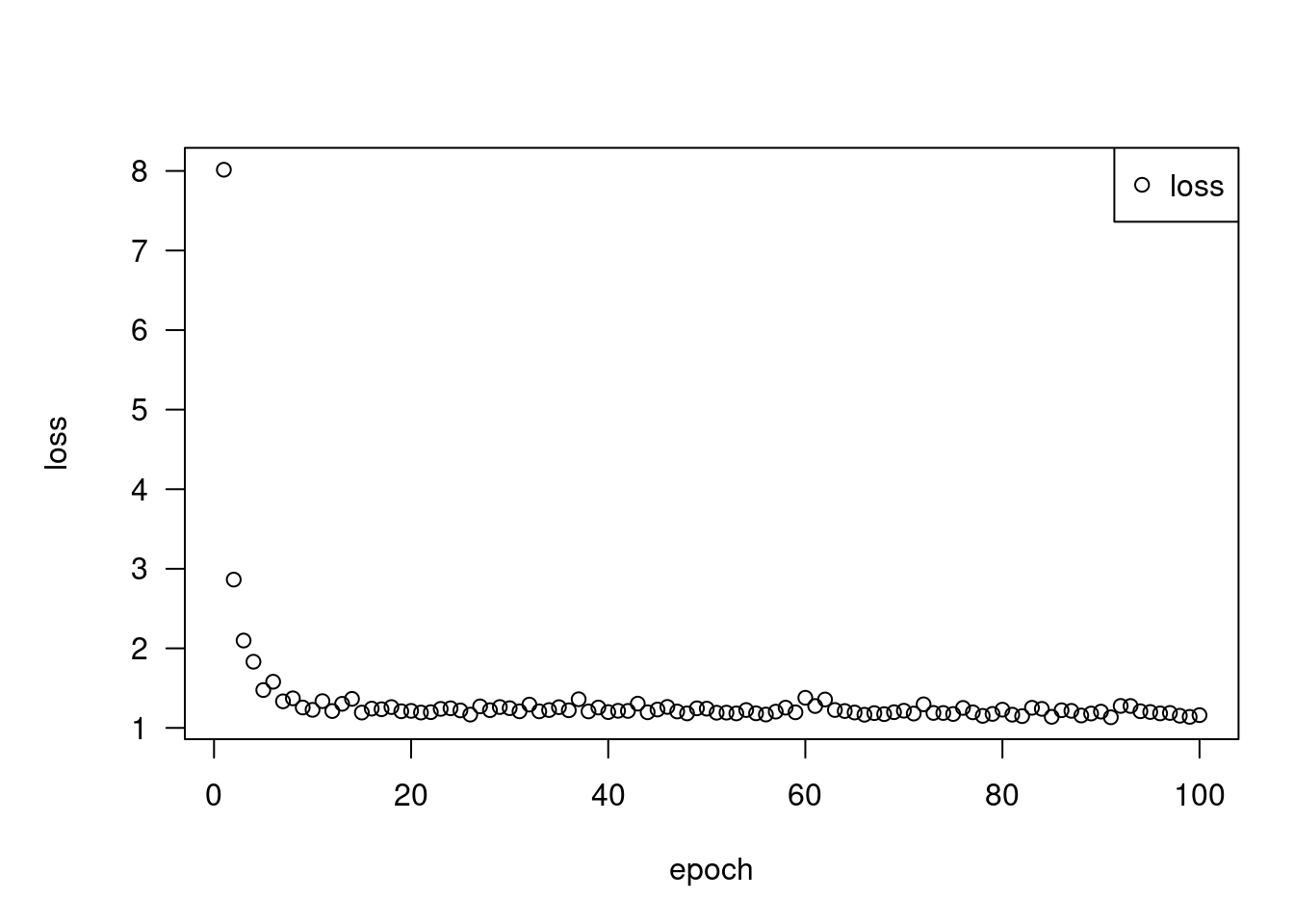# Tensorflow Basics

Start here for a quick overview of TensorFlow basics.

## Tensors

TensorFlow operates on multidimensional arrays or tensors represented as `tensorflow.tensor` objects. Here is a two-dimensional tensor:

``````library(tensorflow)

x <- as_tensor(1:6, dtype = "float32", shape = c(2, 3))

x``````
``````tf.Tensor(
[[1. 2. 3.]
[4. 5. 6.]], shape=(2, 3), dtype=float32)``````
``x\$shape``
``TensorShape([2, 3])``
``x\$dtype``
``tf.float32``

The most important attributes of a tensor are its `shape` and `dtype`:

• `tensor\$shape`: tells you the size of the tensor along each of its axes.
• `tensor\$dtype`: tells you the type of all the elements in the tensor.

TensorFlow implements standard mathematical operations on tensors, as well as many operations specialized for machine learning.

For example:

``x + x``
``````tf.Tensor(
[[ 2.  4.  6.]
[ 8. 10. 12.]], shape=(2, 3), dtype=float32)``````
``5 * x``
``````tf.Tensor(
[[ 5. 10. 15.]
[20. 25. 30.]], shape=(2, 3), dtype=float32)``````
``tf\$matmul(x, t(x)) ``
``````tf.Tensor(
[[14. 32.]
[32. 77.]], shape=(2, 2), dtype=float32)``````
``tf\$concat(list(x, x, x), axis = 0L)``
``````tf.Tensor(
[[1. 2. 3.]
[4. 5. 6.]
[1. 2. 3.]
[4. 5. 6.]
[1. 2. 3.]
[4. 5. 6.]], shape=(6, 3), dtype=float32)``````
``tf\$nn\$softmax(x, axis = -1L)``
``````tf.Tensor(
[[0.09003057 0.24472848 0.6652409 ]
[0.09003057 0.24472848 0.6652409 ]], shape=(2, 3), dtype=float32)``````
``sum(x) # same as tf\$reduce_sum(x)``
``tf.Tensor(21.0, shape=(), dtype=float32)``

Running large calculations on CPU can be slow. When properly configured, TensorFlow can use accelerator hardware like GPUs to execute operations very quickly.

``````if (length(tf\$config\$list_physical_devices('GPU')))
message("TensorFlow **IS** using the GPU") else
message("TensorFlow **IS NOT** using the GPU")``````
``TensorFlow **IS** using the GPU``

Refer to the Tensor guide for details.

## Variables

Normal tensor objects are immutable. To store model weights (or other mutable state) in TensorFlow use a `tf\$Variable`.

``````var <- tf\$Variable(c(0, 0, 0))
var``````
``<tf.Variable 'Variable:0' shape=(3,) dtype=float32, numpy=array([0., 0., 0.], dtype=float32)>``
``var\$assign(c(1, 2, 3))``
``<tf.Variable 'UnreadVariable' shape=(3,) dtype=float32, numpy=array([1., 2., 3.], dtype=float32)>``
``var\$assign_add(c(1, 1, 1))``
``<tf.Variable 'UnreadVariable' shape=(3,) dtype=float32, numpy=array([2., 3., 4.], dtype=float32)>``

Refer to the Variables guide for details.

## Automatic differentiation

Gradient descent and related algorithms are a cornerstone of modern machine learning.

To enable this, TensorFlow implements automatic differentiation (autodiff), which uses calculus to compute gradients. Typically you’ll use this to calculate the gradient of a model’s error or loss with respect to its weights.

``````x <- tf\$Variable(1.0)

f <- function(x)
x^2 + 2*x - 5``````
``f(x)``
``tf.Tensor(-2.0, shape=(), dtype=float32)``

At `x = 1.0`, `y = f(x) = (1^2 + 2*1 - 5) = -2`.

The derivative of `y` is `y' = f'(x) = (2*x + 2) = 4`. TensorFlow can calculate this automatically:

``````with(tf\$GradientTape() %as% tape, {
y <- f(x)
})

g_x <- tape\$gradient(y, x)  # g(x) = dy/dx

g_x``````
``tf.Tensor(4.0, shape=(), dtype=float32)``

This simplified example only takes the derivative with respect to a single scalar (`x`), but TensorFlow can compute the gradient with respect to any number of non-scalar tensors simultaneously.

Refer to the Autodiff guide for details.

## Graphs and `tf_function`

While you can use TensorFlow interactively like any R library, TensorFlow also provides tools for:

• Performance optimization: to speed up training and inference.
• Export: so you can save your model when it’s done training.

These require that you use `tf_function()` to separate your pure-TensorFlow code from R.

``````my_func <- tf_function(function(x) {
message('Tracing.')
tf\$reduce_sum(x)
})``````

The first time you run the `tf_function`, although it executes in R, it captures a complete, optimized graph representing the TensorFlow computations done within the function.

``````x <- as_tensor(1:3)
my_func(x)``````
``Tracing.``
``tf.Tensor(6, shape=(), dtype=int32)``

On subsequent calls TensorFlow only executes the optimized graph, skipping any non-TensorFlow steps. Below, note that `my_func` doesn’t print `"Tracing."` since `message` is an R function, not a TensorFlow function.

``````x <- as_tensor(10:8)
my_func(x)``````
``tf.Tensor(27, shape=(), dtype=int32)``

A graph may not be reusable for inputs with a different signature (`shape` and `dtype`), so a new graph is generated instead:

``````x <- as_tensor(c(10.0, 9.1, 8.2), dtype=tf\$dtypes\$float32)
my_func(x)``````
``Tracing.``
``tf.Tensor(27.3, shape=(), dtype=float32)``

These captured graphs provide two benefits:

• In many cases they provide a significant speedup in execution (though not this trivial example).
• You can export these graphs, using `tf\$saved_model`, to run on other systems like a server or a mobile device, no Python installation required.

Refer to Intro to graphs for more details.

## Modules, layers, and models

`tf\$Module` is a class for managing your `tf\$Variable` objects, and the `tf_function` objects that operate on them. The `tf\$Module` class is necessary to support two significant features:

1. You can save and restore the values of your variables using `tf\$train\$Checkpoint`. This is useful during training as it is quick to save and restore a model’s state.
2. You can import and export the `tf\$Variable` values and the `tf\$function` graphs using `tf\$saved_model`. This allows you to run your model independently of the Python program that created it.

Here is a complete example exporting a simple `tf\$Module` object:

``````library(keras) # %py_class% is exported by the keras package at this time
MyModule(tf\$Module) %py_class% {
initialize <- function(self, value) {
self\$weight <- tf\$Variable(value)
}

multiply <- tf_function(function(self, x) {
x * self\$weight
})
}``````
``````mod <- MyModule(3)
mod\$multiply(as_tensor(c(1, 2, 3), "float32"))``````
``tf.Tensor([3. 6. 9.], shape=(3), dtype=float32)``

Save the `Module`:

``````save_path <- tempfile()
tf\$saved_model\$save(mod, save_path)``````

The resulting SavedModel is independent of the code that created it. You can load a SavedModel from R, Python, other language bindings, or TensorFlow Serving. You can also convert it to run with TensorFlow Lite or TensorFlow JS.

``````reloaded <- tf\$saved_model\$load(save_path)
``tf.Tensor([3. 6. 9.], shape=(3), dtype=float32)``

The `tf\$keras\$layers\$Layer` and `tf\$keras\$Model` classes build on `tf\$Module` providing additional functionality and convenience methods for building, training, and saving models. Some of these are demonstrated in the next section.

Refer to Intro to modules for details.

## Training loops

Now put this all together to build a basic model and train it from scratch.

First, create some example data. This generates a cloud of points that loosely follows a quadratic curve:

``````x <- as_tensor(seq(-2, 2, length.out = 201), "float32")

f <- function(x)
x^2 + 2*x - 5

ground_truth <- f(x)
y <- ground_truth + tf\$random\$normal(shape(201))

x %<>% as.array()
y %<>% as.array()
ground_truth %<>% as.array()

plot(x, y, type = 'p', col = "deepskyblue2", pch = 19)
lines(x, ground_truth, col = "tomato2", lwd = 3)
legend("topleft",
col = c("deepskyblue2", "tomato2"),
lty = c(NA, 1), lwd = 3,
pch = c(19, NA),
legend = c("Data", "Ground Truth"))``````Create a model:

``````Model(tf\$keras\$Model) %py_class% {
initialize <- function(units) {
super\$initialize()
self\$dense1 <- layer_dense(
units = units,
activation = tf\$nn\$relu,
kernel_initializer = tf\$random\$normal,
bias_initializer = tf\$random\$normal
)
self\$dense2 <- layer_dense(units = 1)
}

call <- function(x, training = TRUE) {
x %>%
.[, tf\$newaxis] %>%
self\$dense1() %>%
self\$dense2() %>%
.[, 1]
}
}``````
``model <- Model(64)``
``````untrained_predictions <- model(as_tensor(x))

plot(x, y, type = 'p', col = "deepskyblue2", pch = 19)
lines(x, ground_truth, col = "tomato2", lwd = 3)
lines(x, untrained_predictions, col = "forestgreen", lwd = 3)
legend("topleft",
col = c("deepskyblue2", "tomato2", "forestgreen"),
lty = c(NA, 1, 1), lwd = 3,
pch = c(19, NA),
legend = c("Data", "Ground Truth", "Untrained predictions"))
title("Before training")``````Write a basic training loop:

``````variables <- model\$variables

optimizer <- tf\$optimizers\$SGD(learning_rate=0.01)

for (step in seq(1000)) {

prediction <- model(x)
error <- (y - prediction) ^ 2
mean_error <- mean(error)
})

if (step %% 100 == 0)
message(sprintf('Mean squared error: %.3f', as.array(mean_error)))
}``````
``Mean squared error: 1.214``
``Mean squared error: 1.162``
``Mean squared error: 1.141``
``Mean squared error: 1.130``
``Mean squared error: 1.122``
``Mean squared error: 1.115``
``Mean squared error: 1.110``
``Mean squared error: 1.106``
``Mean squared error: 1.101``
``Mean squared error: 1.098``
``````trained_predictions <- model(x)
plot(x, y, type = 'p', col = "deepskyblue2", pch = 19)
lines(x, ground_truth, col = "tomato2", lwd = 3)
lines(x, trained_predictions, col = "forestgreen", lwd = 3)
legend("topleft",
col = c("deepskyblue2", "tomato2", "forestgreen"),
lty = c(NA, 1, 1), lwd = 3,
pch = c(19, NA),
legend = c("Data", "Ground Truth", "Trained predictions"))
title("After training")``````That’s working, but remember that implementations of common training utilities are available in the `tf\$keras` module. So consider using those before writing your own. To start with, the `compile` and `fit` methods for Keras `Model`s implement a training loop for you:

``new_model <- Model(64)``
``````new_model %>% compile(
loss = tf\$keras\$losses\$MSE,
optimizer = tf\$optimizers\$SGD(learning_rate = 0.01)
)

history <- new_model %>%
fit(x, y,
epochs = 100,
batch_size = 32,
verbose = 0)

model\$save('./my_model')``````
``plot(history, metrics = 'loss', method = "base") ````# see ?plot.keras_training_history for more options.``

Refer to Basic training loops and the Keras guide for more details.

## Environment Details

``tensorflow::tf_config()``
``````TensorFlow v2.11.0 (~/.virtualenvs/r-tensorflow-website/lib/python3.10/site-packages/tensorflow)
Python v3.10 (~/.virtualenvs/r-tensorflow-website/bin/python)``````
``sessionInfo()``
``````R version 4.2.1 (2022-06-23)
Platform: x86_64-pc-linux-gnu (64-bit)
Running under: Ubuntu 20.04.5 LTS

Matrix products: default
BLAS:   /home/tomasz/opt/R-4.2.1/lib/R/lib/libRblas.so
LAPACK: /usr/lib/x86_64-linux-gnu/libmkl_intel_lp64.so

locale:
 LC_CTYPE=en_US.UTF-8       LC_NUMERIC=C
 LC_TIME=en_US.UTF-8        LC_COLLATE=en_US.UTF-8
 LC_MONETARY=en_US.UTF-8    LC_MESSAGES=en_US.UTF-8
 LC_PAPER=en_US.UTF-8       LC_NAME=C
 LC_MEASUREMENT=en_US.UTF-8 LC_IDENTIFICATION=C

attached base packages:
 stats     graphics  grDevices utils     datasets  methods   base

other attached packages:
 keras_2.9.0.9000      tensorflow_2.9.0.9000

loaded via a namespace (and not attached):
 Rcpp_1.0.9             pillar_1.8.1           compiler_4.2.1
 base64enc_0.1-3        tools_4.2.1            zeallot_0.1.0
 digest_0.6.31          jsonlite_1.8.4         evaluate_0.18
 lifecycle_1.0.3        tibble_3.1.8           lattice_0.20-45
 pkgconfig_2.0.3        png_0.1-8              rlang_1.0.6
 Matrix_1.5-3           cli_3.4.1              yaml_2.3.6
 xfun_0.35              fastmap_1.1.0          stringr_1.5.0
 knitr_1.41             generics_0.1.3         vctrs_0.5.1
 htmlwidgets_1.5.4      rprojroot_2.0.3        grid_4.2.1
 reticulate_1.26-9000   glue_1.6.2             here_1.0.1
 R6_2.5.1               tfautograph_0.3.2.9000 fansi_1.0.3
 rmarkdown_2.18         magrittr_2.0.3         whisker_0.4.1
 backports_1.4.1        htmltools_0.5.4        tfruns_1.5.1
 utf8_1.2.2             stringi_1.7.8         ``````
``system2(reticulate::py_exe(), c("-m pip freeze"), stdout = TRUE) |> writeLines()``
``````absl-py==1.3.0
asttokens==2.2.1
astunparse==1.6.3
backcall==0.2.0
cachetools==5.2.0
certifi==2022.12.7
charset-normalizer==2.1.1
decorator==5.1.1
dill==0.3.6
etils==0.9.0
executing==1.2.0
flatbuffers==22.12.6
gast==0.4.0
grpcio==1.51.1
h5py==3.7.0
idna==3.4
importlib-resources==5.10.1
ipython==8.7.0
jedi==0.18.2
kaggle==1.5.12
keras==2.11.0
keras-tuner==1.1.3
kt-legacy==1.0.4
libclang==14.0.6
Markdown==3.4.1
MarkupSafe==2.1.1
matplotlib-inline==0.1.6
numpy==1.23.5
oauthlib==3.2.2
opt-einsum==3.3.0
packaging==22.0
pandas==1.5.2
parso==0.8.3
pexpect==4.8.0
pickleshare==0.7.5
Pillow==9.3.0
promise==2.3
prompt-toolkit==3.0.36
protobuf==3.19.6
ptyprocess==0.7.0
pure-eval==0.2.2
pyasn1==0.4.8
pyasn1-modules==0.2.8
pydot==1.4.2
Pygments==2.13.0
pyparsing==3.0.9
python-dateutil==2.8.2
python-slugify==7.0.0
pytz==2022.6
PyYAML==6.0
requests==2.28.1
requests-oauthlib==1.3.1
rsa==4.9
scipy==1.9.3
six==1.16.0
stack-data==0.6.2
tensorboard==2.11.0
tensorboard-data-server==0.6.1
tensorboard-plugin-wit==1.8.1
tensorflow==2.11.0
tensorflow-datasets==4.7.0
tensorflow-estimator==2.11.0
tensorflow-hub==0.12.0
tensorflow-io-gcs-filesystem==0.28.0
termcolor==2.1.1
text-unidecode==1.3
toml==0.10.2
tqdm==4.64.1
traitlets==5.7.1
typing_extensions==4.4.0
urllib3==1.26.13
wcwidth==0.2.5
Werkzeug==2.2.2
wrapt==1.14.1
zipp==3.11.0``````
``````TF Devices:
-  PhysicalDevice(name='/physical_device:CPU:0', device_type='CPU')
-  PhysicalDevice(name='/physical_device:GPU:0', device_type='GPU')
CPU cores: 12
Date rendered: 2022-12-16
Page render time: 34 seconds``````